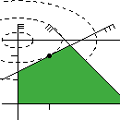CGAL 4.14 - Linear and Quadratic Programming Solver
Linear and Quadratic Programming Solver ReferenceKaspar Fischer, Bernd Gärtner, Sven Schönherr, and Frans Wessendorp
This package contains algorithms for minimizing linear and convex quadratic functions over polyhedral domains, described by linear equations and inequalities. The algorithms are exact, i.e. the solution is computed in terms of multiprecision rational numbers. The resulting solution is certified: along with the claims that the problem under consideration has an optimal solution, is infeasible, or is unbounded, the algorithms also deliver proofs for these facts. These proofs can easily (and independently from the algorithms) be checked for correctness. The solution algorithms are based on a generalization of the simplex method to quadratic objective functions.
Introduced in: CGAL 3.3
BibTeX: cgal:fgsw-lqps-19a

## Concepts

• QuadraticProgram (for quadratic programs with variable bounds l <= x <= u)
• LinearProgram (for linear programs with variable bounds l <= x <= u)
• NonnegativeQuadraticProgram (for quadratic programs with variable bounds x >= 0)
• NonnegativeLinearProgram (for linear programs with variable bounds x >= 0)
• MPSFormat (the format used for reading and writing linear and quadratic programs)

## Classes

There is a class that represents the solution of a linear or quadratic program. An instance of this class is returned by any of the solution functions below.

• CGAL::Quadratic_program_solution<ET>

We offer a number of predefined models for the above program concepts. The following two are simultaneously models for all four concepts and are probably the most convenient models; they allow you to construct linear or quadratic programs entry by entry, or from streams in MPSFormat. At any time, you can query these programs for linearity and nonnegativity and thus select the appropriate solution function.

• CGAL::Quadratic_program<NT> (for linear and quadratic programs that own their data and are built entry-wise)
• CGAL::Quadratic_program_from_mps<NT> (for linear and quadratic programs read from an input stream in MPSFormat; the constructed program can also be manipulate entry-wise)

Then there are specific models for any of the four program concepts above; these are useful if you want to maintain the program data yourself, since they simply wrap random access iterators over the program data and involve no further copying of data.

• CGAL::Quadratic_program_from_iterators<A_it, B_it, R_it, FL_it, L_it, FU_it, U_it, D_it, C_it> (for quadratic programs that wrap given iterators, without copying data)
• CGAL::Linear_program_from_iterators<A_it, B_it, R_it, FL_it, L_it, FU_it, U_it, C_it> (for linear programs wrapping given iterators)
• CGAL::Nonnegative_quadratic_program_from_iterators<A_it, B_it, R_it, D_it, C_it> (for nonnegative quadratic programs, wrapping given iterators)
• CGAL::Nonnegative_linear_program_from_iterators<A_it, B_it, R_it, C_it> (for nonnegative linear programs, wrapping given iterators)

## Functions

In case you want to construct a program from complicated iterators (whose types you don't know, or simply don't want to bother with), we provide four makers.

• CGAL::make_quadratic_program_from_iterators()
• CGAL::make_linear_program_from_iterators()
• CGAL::make_nonnegative_quadratic_program_from_iterators()
• CGAL::make_nonnegative_linear_program_from_iterators()

There are four functions to solve a program, one for each program concept:

• CGAL::solve_quadratic_program()
• CGAL::solve_linear_program()
• CGAL::solve_nonnegative_quadratic_program()
• CGAL::solve_nonnegative_linear_program()

The solution process can customized by passing an object of the class

• CGAL::Quadratic_program_options

Programs can be written to an output stream in MPSFormat, using one of the following four functions:

• CGAL::print_quadratic_program()
• CGAL::print_linear_program()
• CGAL::print_nonnegative_quadratic_program()
• CGAL::print_nonnegative_linear_program()

## Modules

Concepts

Classes
This module provides high and low level classes that allow to construct and represent linear and quadratic programs and their solution.

Functions
This module provides makers to construct a program, as well as functions to solve and print programs.